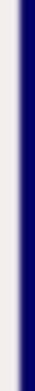homework help forum today's math problem links utilities previous math problem feedback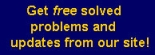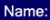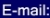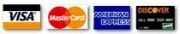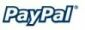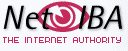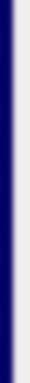# MATH HOMEWORK HELP

16. Use the second derivative test to find the x-coordinates of the relative maximum and the relative minimum points of the following function.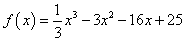Solution: We first find the critical points.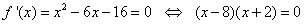Therefore, the critical points are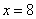and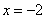. We now compute the second derivative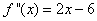Observe that:
•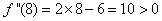. This means thatis a relative minimum
•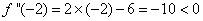. This means thatis a relative maximum.

MGT - Your source of Math and Statistics Homework Help